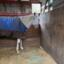Ask your own question, for FREE!
Mathematicskittybasil:

QUERY: word problem? Not sure if this means the entire window or just the labeled portionkittybasil:

I have the answer for a single and the full set of panels in the hexagon.kittybasil:

I apologize for tagging two mods but you guys are the best math professionals I know out there @Vocaloid @angleAngle:

What are you stuck with? Step 1: Solve for the area of 1 of the triangles using the given formula Step 2: Multiply by 6 because there are 6 triangleskittybasil:

Well, I know how to solve the problem, except idk if it's asking for the entire window or not. Wording is unclearAngle:

oh yeah entire windowkittybasil:

Especially since it says "windows" for some reason in the beginning. Extra confusionAngle:

nono you're over thinking it the first sentence is saying that the sunlight for any window depends on the area of the glass of the window in generalAngle:

because it was talking about 'in general' it used plural in the first sentencekittybasil:

Thank you so much! 🏅kittybasil:

Protip for those who are studying/learning this kind of math:

To get a singular panel, use triangular area formula $$A=\frac{1}{2}bh$$ where $$b=6$$ and $$h=5.2$$,$\therefore A_{panel}=\frac{1}{2}(6)(5.2)=\frac{1}{2}{31.2}=15.6\text{ in}^2$
To get the entire window, just multiply the previous value by 6 since there are six panels:$A_{window}=6\cdot A_{panel}=6\cdot15.6\text{ in}^2=93.6\text{ in}^2$
Hope that helped!kittybasil:

I wish I could edit the original question to make it a reference post, but editing is buggedkittybasil:

Actually, I had another question. Would this be $$0.77\overline{87}$$ or $$0.7\overline{78}$$? (Since nobody knows if there's another 8 at the tail end)Angle:

the latter 0.778 with the 78 repeatingAngle:

it gets highlighted for when the pattern first starts repeatingkittybasil:

I thought so too but idk if there's an 8 at the end after wait, highlighted?Angle:

I meant with the line over itkittybasil:

yeah, I wasn't sure which version it was due to not being sure if there is an 8 at the end (which there would be if it's $$0.7\overline{78}$$ right?)Angle:

there is no endkittybasil:

oh I mean 8 at the end of revealed sequence, my badAngle:

it doesn't matter, the dot dot dots mean that whatever pattern you see should be repeatedkittybasil:

ah, okay but is $$0.77\overline{87}$$ still valid though? That was my main issue because both look like they qualify as the answer, but there's only one 💀kittybasil:

one correct answer*Angle:

assume that mathematicians are lazy - always pick the most efficient notationkittybasil:

😂 OkayAngle:

it would be technically correct, but in terms of a multiple choice question - that is how I would think about itkittybasil:

So then go with this - ?$\large{0.7\overline{78}}$Angle:

correctkittybasil:

Okay, ty!! double medals should be allowed - jkAngle:

well you see... this is why we only allow one question per postkittybasil:

oh no I was joking hahakittybasil:

Recap for those who are trying to sort through the thread. Here is a summary of all the content discussed!

kittybasil wrote:
Protip for those who are studying/learning this kind of math: To get a singular panel, use triangular area formula $$A=\frac{1}{2}bh$$ where $$b=6$$ and $$h=5.2$$,$\therefore A_{panel}=\frac{1}{2}(6)(5.2)=\frac{1}{2}{31.2}=15.6\text{ in}^2$ To get the entire window, just multiply the previous value by 6 since there are six panels:$A_{window}=6\cdot A_{panel}=6\cdot15.6\text{ in}^2=93.6\text{ in}^2$
Also, recap of the second question: $$0.778787...$$ should translate to this:$\large{0.7\overline{78}}$

Can't find your answer? Make a FREE account and ask your own questions, OR help others and earn volunteer hours!

Join our real-time social learning platform and learn together with your friends!
Latest Questionsmarissabartoldo: help
16 minutes ago 3 Replies 1 Medalilightnight64: new visualizer hope yall like it : D song: just like you https://vizzy.io/project
22 minutes ago 0 Replies 0 Medalsmarissabartoldo: help
23 minutes ago 5 Replies 1 MedalUmmWhat: Song: https://www.youtube.com/watch?v=1ohvhmGSfxI
36 minutes ago 4 Replies 3 Medalstkemp25: A satellite launch rocket had a cylindrical fuel tank.the fuel tank can hold v cubic meters of fuel.
1 hour ago 1 Reply 1 MedalTrapmya: Can someone please explain this to me work is below
1 hour ago 9 Replies 1 Medalmaddie123: 1
7 minutes ago 7 Replies 0 Medalsmaddie123: A number is divided by 6. Then, 3 is added to the quotient, and the result is 5. What is the number? * A.
2 hours ago 6 Replies 0 MedalsUmmWhat: A pile of 15 boxes is 3 meters high. What is the depth of each box?
1 hour ago 9 Replies 1 Medalmaddie123: help
2 hours ago 2 Replies 0 Medals
Can't find your answer? Make a FREE account and ask your own questions, OR help others and earn volunteer hours!

Join our real-time social learning platform and learn together with your friends!